# 机器学习-吴恩达：学习笔记及总结（3）

## Coursera | Machine Learning | Andrew Ng

Thistledown    March 4, 2020

Course Link ：Week 3 - Logistic Regression

## 7 Classification and Representation

#### 7.1 Classification

• The classification problem is just like the regression problem, except that the values we now want to predict take on only a small number of discrete values.
• For now, we will focus on the binary classification problem.

• $y \in {0,1}$ - the variable that we’re trying to predict
• $0: \text{“Negative Class”}$ (e.g., benign tumor)
• $1: \text{“Positive Class”}$ (e.g., malignant tumor)
• Applying linear regression to a classification problem often isn’t a great idea.
• Logistic Regression: $0\leq h_\theta(x) \leq 1$ (BTW, this is actually a classification algorithm)

#### 7.2 Hypothesis Representation

• Logistic Regression Model: want $0 \leq h_\theta(x) \leq 1$
• $h_\theta(x) = g(\theta^Tx)$
• Sigmoid function (or logistic function): $g(z) = \frac{1}{1 + e^{-z}}$. The following image shows us what the sigmoid function looks like: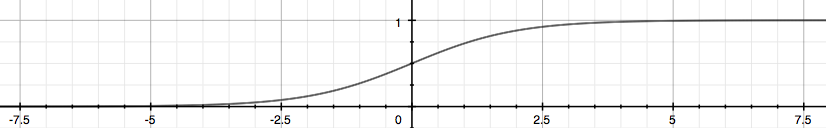• $h_\theta(x) = \frac{1}{1+e^{-\theta^Tx}}$
• Interpretation of hypothesis output:
• $h_\theta(x) = \text{estimated probability that } y=1 \text{ on input }x$
• $h_\theta(x)= P(y=1 \vert x;\theta)$ : probability that $y = 1$, given $x$, parameterized by $\theta$
• $P(y=0 \vert x;\theta) + P(y=1 \vert x;\theta) = 1 \\ P(y=0 \vert x;\theta) = 1 - P(y=1 \vert x;\theta)$

#### 7.3 Decision Boundary

• Logistic regression: $h_\theta(x) = g(\theta^Tx), \quad g(z)=\frac{1}{1+e^{-z}}$
• Suppose predict $y=\begin{cases} 0, & \text {if$h_\theta(x) \geq$0.5} \newline 1, & \text{if$h_\theta(x) < $0.5} \end{cases}$
• Decision boundary: the decision boundary is the line that separates the area where $y=0$ and where $y=1$.
• It is created by our hypothesis function
• The input to the sigmoid function of $g(z)$ doesn’t need to be linear, and could be a function that describes a circle or any shape to fit the data

## 8 Logistic Regression Model

#### 8.1 Cost Function

• Logistic regression cost function:
• $\text{Cost}(h_\theta(x), y)=\begin{cases} -\log(h_\theta(x)), & \text {if } y =1 \newline -\log(1-h_\theta(x)), & \text{if } y=0 \end{cases}$
• $\text{Cost}(h_\theta(x), y) = 0, \text{ if } h_\theta(x) = y$
• $\text{Cost}(h_\theta(x), y) \rightarrow \infty, \text{ if$y=0$and$h_\theta(x) \rightarrow 1$}$
• $\text{Cost}(h_\theta(x), y) \rightarrow \infty, \text{ if$y=1$and$h_\theta(x) \rightarrow 0$}$
• When $y=1$, we get the following plot for $J(\theta) \text{ vs } h_\theta(x)$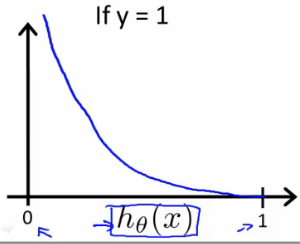• Similarly, when $y=0$, we get the following plot for $J(\theta) \text{ vs } h_\theta(x)$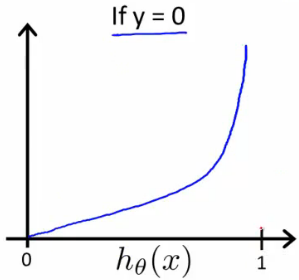#### 8.2 Simplified Cost Function and Gradient Descent

• Logistic regression cost function

• $J(\theta) = \frac{1}{m}\sum_{i =1}^{m}\text{Cost}(h_\theta(x^{(i)}, y^{(i)} )$
• $\text{Cost}(h_\theta(x), y)=\begin{cases} -\log(h_\theta(x)), & \text {if } y =1 \newline -\log(1-h_\theta(x)), & \text{if } y=0 \end{cases}$
• $\text{Note:$y=0$or$ 1$always}$
• Then, $\text{Cost}(h_\theta(x), y)=-y\log(h_\theta(x)) - (1-y)\log(1-h_\theta(x))$

• A vectorized implementation is:

• $h = g(X\theta)$
• $J(\theta) = \frac{1}{m}\cdot \left(-y^T\log(h)-(1-y)^T\log(1-h)\right)$
• To fit parameters $\theta : \mathop{\text{min}}\limits_\theta J(\theta)$

• To make a prediction given new $x$ : output $h_\theta(x) = \frac{1}{1+e^{-\theta^Tx}}$

(Algorithm looks identical to linear regression!)

• A vectorized implementation is: $\theta := \theta - \frac{\alpha}{m}X^T\left( g(X\theta)- \vec{y} \right)$

• Cost function $J(\theta)$. Want $\mathop{\text{min}}\limits_\theta J(\theta)$.

• Given $\theta$, we have code that can compute

• $J(\theta)$
• $\frac{\partial}{\partial \theta_j}J(\theta), \ \text{(for$j = 0, 1, \dots,n$)}$
• Optimization algorithms:

• (In this class) Gradient descent
• No need to manually pick $\alpha$
• Often faster that gradient descent
• More sophisticated

## 9 Multiclass classification

#### 9.1 Multiclass Classification: One-vs-all

• $y = \lbrace 0, 1, \dots, n \rbrace$

• The following image shows how one could classify 3 classes: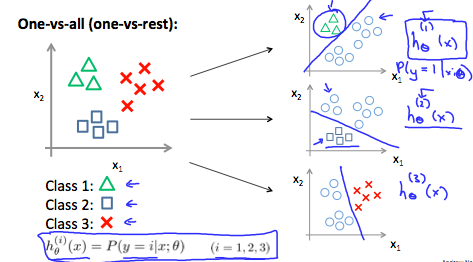• One-vs-all (one-vs-rest):

• $h_\theta^{(i)} = P(y=i \vert x;\theta)\quad (i=1,2,\dots,n)$
• Train a logistic regression classifier $h_\theta^{(i)}(x)$ for each class $i$ to predict the probability that $y = i$.
• On a new input $x$, to make a prediction, pick the class i that maximizes $h_\theta(x)$ : $\mathop{\text{max}}\limits_i h_\theta^{(i)}(x)$

## 10 Solving the Problem of Overfitting

#### 10.1 The Problem of Overfitting

• Overfitting: If we have too many features, the learned hypothesis may fit the training set well ($J(\theta) = \frac{1}{2m}\sum_{i=1}^{m}\left( h_\theta(x^{(i)}-y^{(i)}) \right)^2\approx 0$), but fail to generalize to new new examples (predict prices on new examples).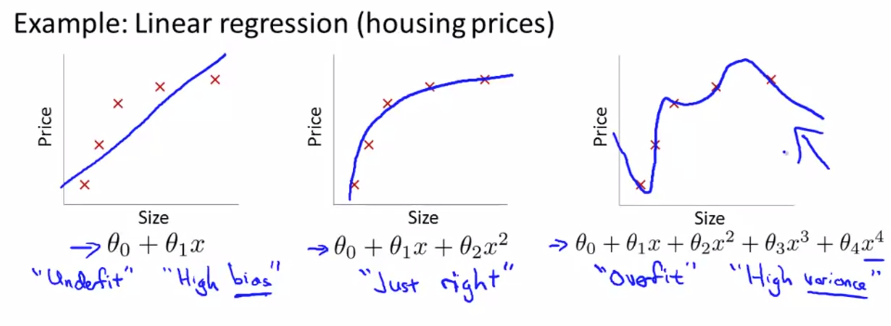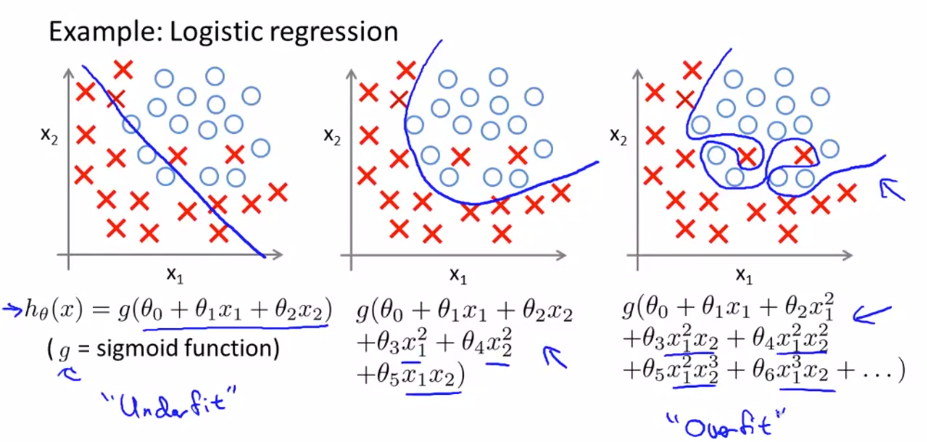• Reduce number of features
• Manually select which features to keep
• Model selection algorithm
• Regularization
• Keep all the features, but reduce magnitude/values of parameters $\theta_j$
• Regularization works well when we have a lot of slightly useful features, each of which contributes a bit to predicting $y$

#### 10.2 Cost Function

• Regularization: small values for parameters $\theta_0, \theta_1, \dots, \theta_n$

• “Simpler” hypothesis
• Less prone to overfitting
• Cost function after regularization:

• $\lambda: \text{regularization parameter}$

#### 10.3 Regularized Linear Regression

• When $j=1, 2, \dots, n$, you can also write $\theta_j := \theta_j(1-\alpha\frac{\lambda}{m}) - \alpha \frac{1}{m} \sum_{i=1}^m\left(h_\theta(x^{(i)})-y^{(i)}\right)x_j^{(i)}$
• $1-\alpha\frac{\lambda}{m} < 1$
• Normal equaiton

• Non-invertibility

• Suppose $\mathop{m}\limits_{\text{#examples}} \leq \mathop{n}\limits_{\text{#features}}$, then $X^TX$ will be non-invertible/singluar.
• $\text{If } \lambda > 0, \ \theta=\left(\underbrace{X^TX+\lambda \begin{bmatrix} 0 & \newline & I_n \end{bmatrix} }_{\text{invertible}} \right)^{-1}X^Ty$

#### 10.4 Regularized Logistic Regression

• We can regularize logistic regression in a similar way that we regularize linear regression. As a result, we can avoid overfitting. The following image shows how the regularized function, displayed by the pick line, is less likely to overfit than the non-regularized function represented by the blue line: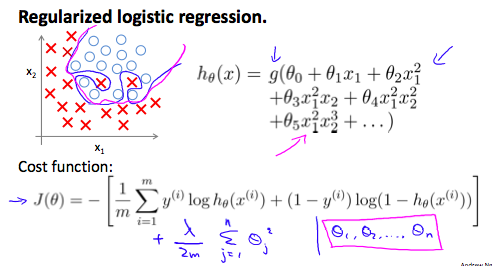• Regularized cost function:

• $\sum_{j=1}^{n}\theta_j^2$ means to explicitly exclude the bias term, $\theta_0$.

## Ex2: Logistic Regression👨‍💻

See this exercise on Coursera-MachineLearning-Python/ex2/ex2.ipynb

#### Ex2.1 Logistic Regression

Instruction: In this part of the exercise, you will build a logistic regression model to predict whether a student gets admitted into a university.

Code:

1
2
3
4
5
6
7
8
9
10
11
12
13
14
15
16
17
18
19
20
21
22
23
24
25
26
27
28
29
30
31
32
33
34
35
36
37
38
39
40
41
42
43
44
45
46
47
48
49
50
51
52
53
54
55
56
57
58
59
60
61
62
63
64
65
66
67
68
69
70
71
72
73
74
75
76
77
78
79
80
81
82
83
84
85
86
87
88
89
90
91
92
93
94
95
import numpy as np
import matplotlib.pyplot as plt
import scipy.optimize as op

# Hypothesis function
def h(X, theta):
return 1 / (1 + np.exp(-np.dot(X, theta)))

# Logistic regression cost function
def costFunction(theta, X, y):
m = len(y)
cost = - np.sum(y * np.log(h(X, theta)) + (1 - y) * np.log(1 - h(X, theta))) / m
grad = np.dot(X.T, h(X, theta) - y) / m

# The objective function to be minimized
def costFunc(params, *args):
X, y = args
[m, n] = X.shape
theta = params.reshape([n, 1])
cost = - np.sum(y * np.log(h(X, theta)) + (1 - y) * np.log(1 - h(X, theta))) / m
return cost

# Method for computing the gradient vector
X, y = args
[m, n] = X.shape
theta = params.reshape([n, 1])
grad = np.dot(X.T, h(X, theta) - y) / m

# The first two columns contains the exam scores and the third column contains the label
X, y = data[:, 0:2], data[:, 2:3]

''' Part 1: Plotting '''
fig0, ax0 = plt.subplots()
label1, label0 = np.where(y.ravel() == 1), np.where(y.ravel() == 0)
ax0.scatter(X[label1, 0], X[label1, 1], marker='+', color='g', label='Admitted')
ax0.scatter(X[label0, 0], X[label0, 1], marker='x', color='r', label='Not admitted')
ax0.legend(loc='upper right')
ax0.set_xlabel('Exam 1 Score')
ax0.set_ylabel('Exam 2 Score')

''' Part 2: Compute Cost and Gradient '''
[m, n] = X.shape
X = np.c_[np.ones([m, 1]), X]           # Add intercept term to x and X_test
initial_theta = np.zeros([n + 1, 1])    # Initialize fitting parameters

# Compute and display initial cost and gradient
cost, grad = costFunction(initial_theta, X, y)

print('\nCost at initial theta : ', cost.ravel())
print('Expected cost (approx): 0.693')
print('Expected gradients (approx): \t-0.1000\t -12.0092\t -11.2628')

# Compute and display cost and gradient with non-zero theta
test_theta = np.array(([-24], [0.2], [0.2]))
cost, grad = costFunction(test_theta, X, y)

print('\nCost at test theta : ', cost.ravel())
print('Expected cost (approx): 0.218')
print('Expected gradients (approx): \t0.043\t 2.5662\t 2.647')

''' Part 3: Optimizing using fminunc '''
params = np.zeros([n + 1, 1])
args = (X, y)

# uUse Newton Conjugate Gradient algorithm to obtain the optimal theta
res = op.minimize(fun=costFunc, x0=params, args=args, method='TNC', jac=gradFunc)
cost, theta = res.fun, res.x

print('\nCost at theta found by fminunc: ', cost)
print('Expected cost (approx): 0.203')
print('theta: ', theta)
print('Expected theta (approx): \t-25.161\t 0.206\t 0.201')

# Plot boundary
x1 = np.arange(min(X[:, 1]), max(X[:, 1]), 1)
x2 = (-theta - theta * x1) / theta
plt.plot(x1, x2, color='blue')
plt.show()

''' Part 4: Predict and Accuracies '''
prob = h(np.array(([1, 45, 85])), theta)
print('\nFor a student with scores 45 and 85, we predict an adimission probability of ', prob)
print('Expected value: 0.775 +/- 0.002')

p = np.where(h(X, theta) > 0.5, 1.0, 0.0)
print('Train accuracy: ', np.mean(p == y.flatten()) * 100, '%')
print('Expected accuracy (approx): 89.0 %\n')


Output:

• Console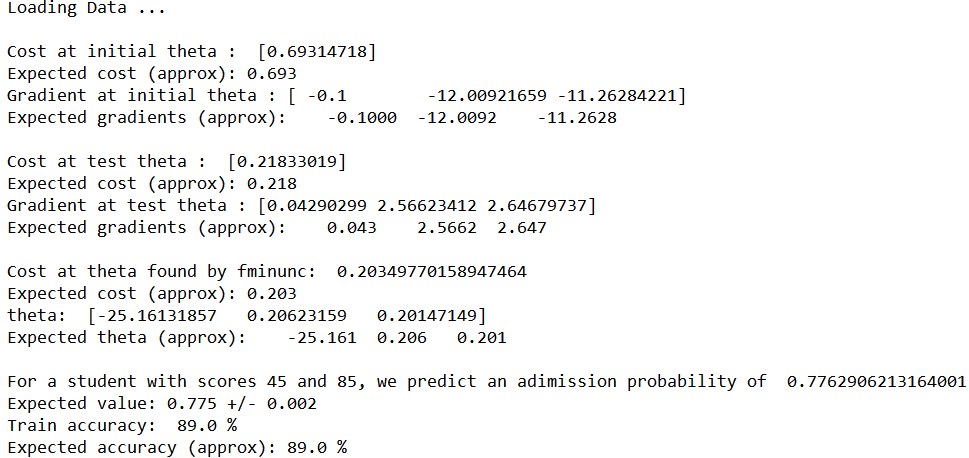• Training data with decision boundary#### Ex2.2 Regularized Logistic Regression

Instruction: In this part of the exercise, you will implement regularized logistic regression to predict whether microchips from a fabrication plant passes quality assurance (QA). During QA, each microchip goes through various tests to ensure it is functioning correctly.

Code:

1
2
3
4
5
6
7
8
9
10
11
12
13
14
15
16
17
18
19
20
21
22
23
24
25
26
27
28
29
30
31
32
33
34
35
36
37
38
39
40
41
42
43
44
45
46
47
48
49
50
51
52
53
54
55
56
57
58
59
60
61
62
63
64
65
66
67
68
69
70
71
72
73
74
75
76
77
78
79
80
81
82
83
84
85
86
87
88
89
90
91
92
93
94
95
96
97
98
99
100
101
102
import numpy as np
import matplotlib.pyplot as plt
from sklearn.preprocessing import PolynomialFeatures

# Feature mapping function to polynomial features
def mapFeature(X1, X2):
degree = 6
X = np.ones([len(X1), 1])
for i in np.arange(1, degree + 1, 1):
for j in range(i + 1):
X = np.c_[X, X1**(i-j) * X2**(j)]
return X

# Hypothesis function
def h(X, theta):
return 1 / (1 + np.exp(-np.dot(X, theta)))

# Compute cost and gradient for logistic regression with regularization
def costFunctionReg(theta, X, y, reg_param):
m = len(y)
cost1 = - np.sum(y * np.log(h(X, theta)) + (1 - y) * np.log(1 - h(X, theta))) / m
cost2 = 0.5 * reg_param * np.dot(theta[1:].T, theta[1:]) / m    # Don't penalize theta_0
cost = cost1 + cost2
grad = np.dot(X.T, h(X, theta) - y) / m
grad[1:] += (reg_param * theta / m)[1:]

# Use Batch Gradient Descent algorithm to minimize cost
def batchGradientDescent(X, y, theta, alpha=0.1, iters = 2000, reg=1):
J_history = np.zeros(iters)
for i in range(iters):
cost, grad = costFunctionReg(theta, X, y, reg)
theta = theta - alpha * grad
J_history[i] = cost
return theta, J_history

# The first two columns contains the exam scores and the third column contains the label
X, y = data[:, 0:2], data[:, 2:3]

# Plot data
fig0, ax0 = plt.subplots()
label1, label0 = np.where(y.ravel() == 1), np.where(y.ravel() == 0)
ax0.scatter(X[label1, 0], X[label1, 1], marker='+', color='k', label='y = 1')
ax0.scatter(X[label0, 0], X[label0, 1], marker='x', color='y', label='y = 0')

''' Part 1: Regularized Logistic Regression '''
m = len(y)
X = mapFeature(X[:, 0], X[:, 1])

# Initialize fitting parameters
initial_theta = np.zeros([X.shape, 1])

# Set regularization parameter lambda to 1
reg_param = 1

# Compute and display inital cost gradient for regularized logistic regression
cost0, grad0 = costFunctionReg(initial_theta, X, y, reg_param)
print('\nCost at initial theta (zeros): ', cost0.flatten())
print('Expected cost (approx): 0.693')
print('Gradient at initial theta (zeros) - first five values only: '
print('Expected gradients (approx) - first five values only: ',
'0.0085 0.0188 0.0001 0.0503, 0.0115')

# Compute and display cost and gradient with all-ones theta and lambda = 10
test_theta = np.ones([X.shape, 1])
cost1, grad1 = costFunctionReg(test_theta, X, y, reg_param=10)

print('\nCost at test theta (with lambda = 10): ', cost1.flatten())
print('Expected cost (approx): 3.16')
print('Gradient at test theta - first five values only: '
print('Expected gradients (approx) - first five values only: ',
'0.3460 0.1614 0.1948 0.2269, 0.0922')

''' Part 2: Regularization and Accuracies '''
# Optimize
theta, J_history = batchGradientDescent(X, y, initial_theta, reg=reg_param)
# fig1, ax1 = plt.subplots()
# ax1.plot(np.arange(2000), J_history, 'c')

# Plot boundary
poly = PolynomialFeatures(6)
x1min, x1max, x2min, x2max = X[:, 1].min(), X[:, 1].max(), X[:, 2].min(), X[:, 2].max()
xx1, xx2 = np.meshgrid(np.linspace(x1min, x1max), np.linspace(x2min, x2max))
bd = 1 / (1 + np.exp(-poly.fit_transform(np.c_[xx1.ravel(), xx2.ravel()]).dot(theta)))
bd = bd.reshape(xx2.shape)
CS = ax0.contour(xx1, xx2, bd, [0.5], colors='c')
CS.collections.set_label('Decision\nBoundary')
ax0.set_title(r'$\lambda$ = '+str(reg_param))
ax0.legend(loc='upper right')
ax0.set_xlabel('Microchip Test 1')
ax0.set_ylabel('Microchip Test 2')
plt.show()

# Compute accuracy on our training set
p = np.where(h(X, theta) >= 0.5, 1.0, 0.0)
print('\nTrain Accuracy: ', np.mean(p == y) * 100, '%')
print('Expected accuracy (with lambda = 1): 83.1 % (approx)')


Output:

• Console ((λ = 1)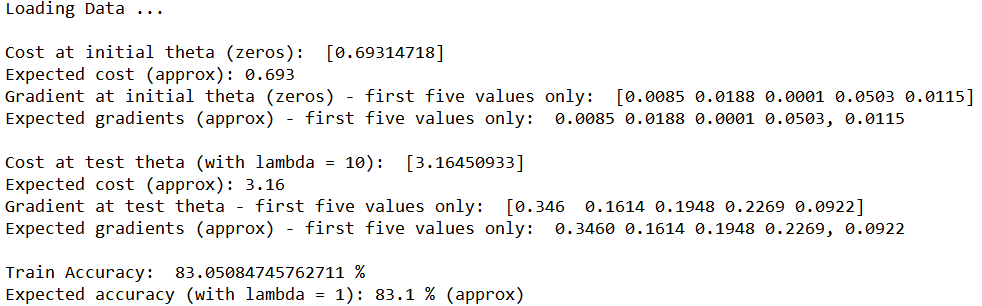• Training data with decision boundary (λ = 1)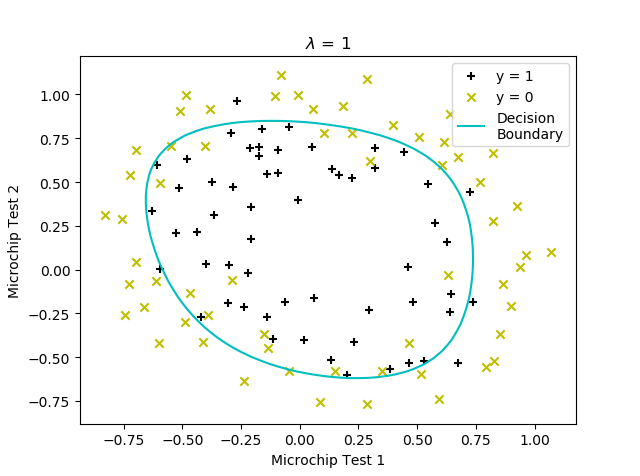• Too much regularization (Underfitting) (λ = 100)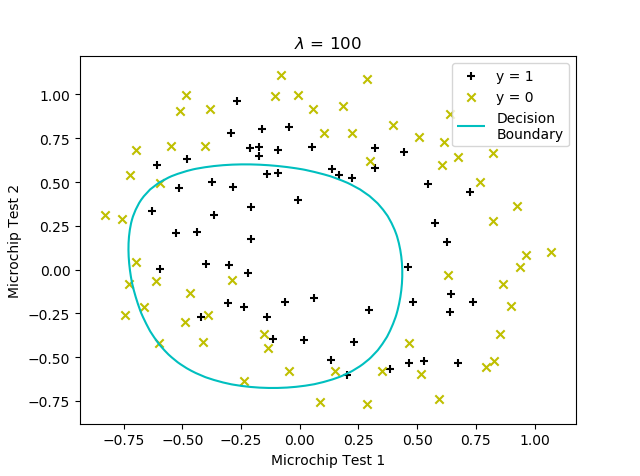Thistledown